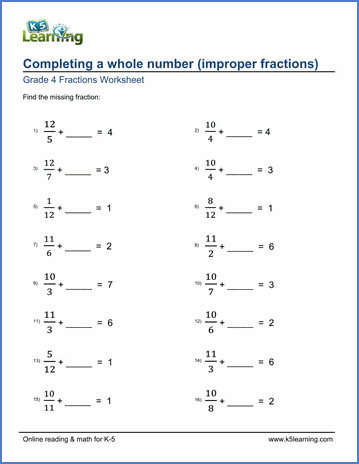# Completing a whole number

## Worksheets: Completing a whole number (adding improper fractions)

Below are six versions of our grade 4 fractions worksheet on adding improper fractions in order to complete a whole number.  Students must find the fraction missing from the addition equation; all sums are whole numbers between 1 and 10.  Answers are expressed as proper or improper fractions without simplification so as to emphasize the concept of 'completing the whole'. These worksheets are pdf files.## More fractions worksheets

Explore all of our fractions worksheets, from dividing shapes into "equal parts" to multiplying and dividing improper fractions and mixed numbers.

## What is K5?

K5 Learning offers reading and math worksheets, workbooks and an online reading and math program for kids in kindergarten to grade 5.  We help your children build good study habits and excel in school.